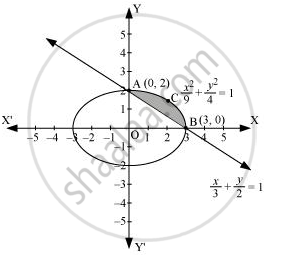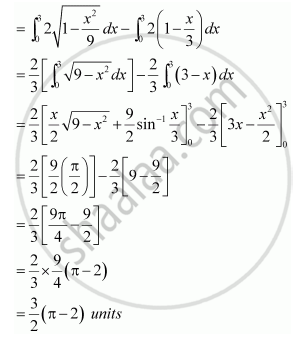Share

# Find the Area of the Smaller Region Bounded by the Ellipse X^2/9 + Y^2/4 And the Line X/3 + Y/2 = 1 - CBSE (Commerce) Class 12 - Mathematics

#### Question

Find the area of the smaller region bounded by the ellipse x^2/9 + y^2/4 and the line x/3 + y/2 = 1

#### Solution

The area of the smaller region bounded by the ellipse, x^2/9 + y^2/4 , and the line, x/3 + y/2 = 1, is represented by the shaded region BCAB as∴ Area BCAB = Area (OBCAO) – Area (OBAO)Is there an error in this question or solution?

#### APPEARS IN

NCERT Solution for Mathematics Textbook for Class 12 (2018 to Current)
Chapter 8: Application of Integrals
Q: 8 | Page no. 375
Solution Find the Area of the Smaller Region Bounded by the Ellipse X^2/9 + Y^2/4 And the Line X/3 + Y/2 = 1 Concept: Area Under Simple Curves.
S